### Home > PC > Chapter 12 > Lesson 12.2.2 > Problem12-52

12-52.
1. The linear transformation M is described by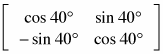. Homework Help ✎

1. Describe the effect of M on a point p = (x, y).

2. Geometrically describe M.

3. Find M −1.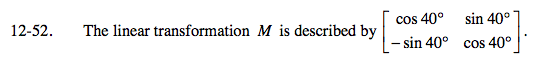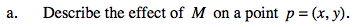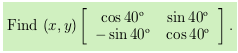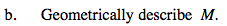Try transforming the points (1, 0) and (0, 1). Where do they end up in relation to where they started?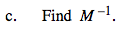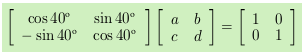Complete the multiplication and solve for a, b, c, and d.